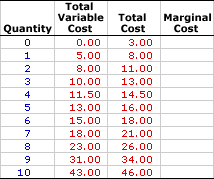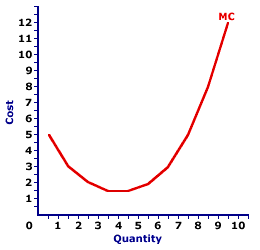Tuesday  March 21, 2023
 AmosWEB means Economics with a Touch of Whimsy!YIELD CURVE: A curve plotting the yields (or returns) on securities with different maturity lengths. The standard yield is for U.S. Treasury securities with lengths ranging from 90 days to 30 years. The five maturity lengths are usually 90 day, 180 day, 2 year, 5 year, 10 year, and 30 year. The shape and slope fo the yield curve indicates the state of the economy and what's likely to come. A normal yield curve has a slight positive slope, with slightly higher yields for longer maturity securities. A steep yield curve suggests the end of a contraction and beginning of an expansion. An inverted, or negatively sloped yield curve is the sign of an upcoming contraction.MARGINAL COST:

The change in total cost (or total variable cost) resulting from a change in the quantity of output produced by a firm in the short run. Marginal cost (MC) indicates how much total cost changes for a given change in the quantity of output. Because changes in total cost are matched by changes in total variable cost in the short run (total fixed cost is fixed), marginal cost is the change in either total cost or total variable cost. It is found by dividing the change in total cost (or total variable cost) by the change in output. Marginal cost is one of four cost concepts used in short-run production analysis. The other three are average total cost, average fixed cost, and average variable cost.
Marginal cost (MC), the change in total cost or total variable cost due to a change in output, is the focal point in the analysis of short-run production cost and how this helps explain the law of supply and the upward-sloping supply curve. While other cost-related terms, including total cost, total variable cost, average total cost, and average variable cost, are noteworthy, marginal cost is without question the most important.

The reason is that increasing marginal cost reflects the law of diminishing marginal returns. As marginal returns decline, marginal cost increases. However, as marginal cost increases, the price a firm needs to receive also increases. The result is a positive relation between price and quantity supplied, which is the law of supply and the supply curve.

Marginal cost tends to be relatively high but declining for small quantities of production. It then reaches a minimum and rises for larger quantities of output. When plotted in a graph, marginal cost traces out a U-shaped pattern. The reason for this shape is directly related to increase and decreasing marginal returns and especially the law of diminishing marginal returns.

The enormous importance of marginal cost to a firm's short-run production decision cannot be overstated. A profit-maximizing firm compares the marginal revenue received from output sold with the marginal cost of producing it. If marginal revenue equals marginal cost, then the firm produces the profit-maximizing output quantity. If marginal revenue is less than marginal cost, then it can boost profit by increasing production. If marginal revenue is greater than marginal cost, then it can boost profit by decreasing production.

### Marginal Cost Equations

Here is the first of two formulas for calculating marginal cost:

 marginal cost = change in total costchange in quantity of output

Because any change in total cost is also an equivalent change in total variable cost (fixed cost DOES NOT change), marginal cost can be calculated using total variable cost, too:

 marginal cost = change in total variable costchange in quantity of output

### Stuffed Animal Cost

Marginal CostConsider a few marginal cost numbers based on the cost of producing Wacky Willy Stuffed Amigos presented in this table. The first column is the quantity of Stuffed Amigos produced per hour, ranging from 0 to 10. The second column is the total variable cost of producing each quantity, ranging from \$0 to \$43. The third column is the total cost of producing each quantity, ranging from \$3 to \$46. The empty fourth column is there to display the marginal cost of producing Stuffed Amigos.

The goal is to fill out this fourth column. To do so, the change in total cost (or total variable cost) attributable to incremental changes in quantity needs to be computed. The first entry in the column is generated by answering the question: How much does total cost (or total variable cost) change when the first Stuffed Amigo is produced? Total cost increases from \$3 to \$8 with the production of the first Stuffed Amigo, a marginal cost of \$5. Similarly total variable cost increases from \$0 to \$5 with the first Stuffed Amigo, also a marginal cost of \$5. Click the [First] button to enter the first marginal cost value in the fourth column.

The marginal cost of producing the second Stuffed Amigo is calculated in the same way. Total cost changes from \$8 to \$11 and total variable cost changes from \$5 to \$8, both generating a marginal cost of \$3. Click the button labeled "Second" to reveal this entry in the table. A click of the [Third] button reveals that the marginal cost of producing the third Stuffed Amigo is \$2, a change in total cost from \$11 to \$13 and a change in total variable cost from \$8 to \$10. To display the entire list of marginal cost values, click the [Remaining] button.

With the table filled, a little interpretation of the numbers seems in order.

• First, marginal cost is relatively high for the first Stuffed Amigo produced, then declines. However, it reaches a low, then rises with the production of additional Stuffed Amigos. The range of values with declining marginal cost is associated with Stage I of production and increasing marginal returns. The range of values with rising marginal cost is associated with Stage II of production, decreasing marginal returns, and the law of diminishing marginal returns. This second range is the more important of the two for the analysis of a firm's profit-maximizing short-run production.

• Second, marginal cost remains positive, it never reaches a zero value let alone negative. The only way for negative marginal cost is for a decrease in total cost, which just does not happen in a real world filled with scarcity, limited resources, unlimited wants and needs, and opportunity cost.

### The Marginal Cost Curve

Marginal Cost CurveThe relation between marginal cost and the quantity of production can be represented by a curve, such as the one conveniently presented in the exhibit to the right.

The key feature of this marginal cost curve is the shape. It is U-shaped, meaning it has a negative slope for small quantities of output, reaches a minimum value, then has a positive slope for larger quantities.

The U-shape of the marginal cost curve is directly attributable to increasing, then decreasing marginal returns (and the law of diminishing marginal returns). As marginal product (and marginal returns) increases for relatively small output quantities, marginal cost declines. Then as marginal product (and marginal returns) decreases with the law of diminishing marginal returns for relatively larger output quantities, marginal cost increases.

### Slope and Total Cost

Marginal cost is the slope of the total cost curve. Because the total cost curve is parallel to the total variable cost curve, marginal cost is also the slope of the total variable cost curve.

This captures the basic mathematical relation that exists between any marginal and the corresponding total. A marginal is always the slope of the total curve. If the total cost curve has a positive slope (that is, is upward sloping), then marginal cost is positive. If the total cost curve has a positive and increasingly steeper slope, then the marginal cost is positive and rising. If the total cost curve has a positive and decreasingly steeper slope, then the marginal cost is positive but falling. This applies in a like manner to total variable cost.

 <= MARGINAL BENEFIT OF SEARCH MARGINAL COST AND LAW OF DIMINISHING MARGINAL RETURNS =>Recommended Citation:

MARGINAL COST, AmosWEB Encyclonomic WEB*pedia, http://www.AmosWEB.com, AmosWEB LLC, 2000-2023. [Accessed: March 21, 2023].

Check Out These Related Terms...

Or For A Little Background...

And For Further Study...
Search Again?WHITE GULLIBON[What's This?] Today, you are likely to spend a great deal of time going from convenience store to convenience store trying to buy either a set of tires or a birthday gift for your grandfather. Be on the lookout for telephone calls from former employers.Your Complete ScopeHelping spur the U.S. industrial revolution, Thomas Edison patented nearly 1300 inventions, 300 of which came out of his Menlo Park "invention factory" during a four-year period."When the solution is simple, God is answering."-- Albert EinsteinTPTotal ProductA PEDestrian's Guide Xtra CreditTell us what you think about AmosWEB. Like what you see? Have suggestions for improvements? Let us know. Click the User Feedback link.| | | | | | | | | | |
| | | |

Thanks for visiting AmosWEB